﻿ Introduction to Polygons
INTRODUCTION TO POLYGONS

DEFINITION polygon
A polygon is a closed figure in a plane made by joining line segments,
where each line segment intersects exactly two others.

Note: Strictly speaking, a polygon does not include its interior (the space inside the polygon).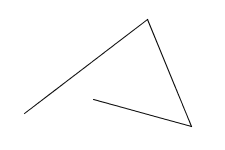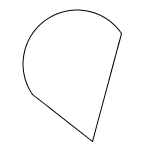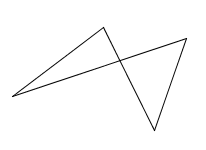a polygon not closed;not a polygon not made of line segments;not a polygon line segment intersects more than two others;not a polygon

Polygons are usually classified according to how many sides they have:

DEFINITIONS names of polygons with various numbers of sides
 A triangle is a polygon with $\,3\,$ sides.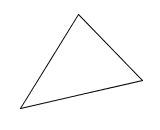A heptagon is a polygon with $\,7\,$ sides.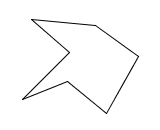A quadrilateral is a polygon with $\,4\,$ sides.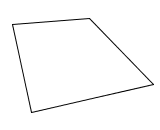An octagon is a polygon with $\,8\,$ sides.A pentagon is a polygon with $\,5\,$ sides.A nonagon is a polygon with $\,9\,$ sides.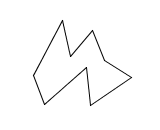A hexagon is a polygon with $\,6\,$ sides.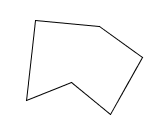A decagon is a polygon with $\,10\,$ sides.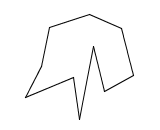More generally, a polygon with $\,n\,$ sides can be called an $\,n\,$-gon.

For example, a polygon with $\,27\,$ sides can be called a $\,27$-gon.

DEFINITION vertex of a polygon; vertices
The vertices of a polygon are the points where its sides intersect.
The singular form of vertices is vertex.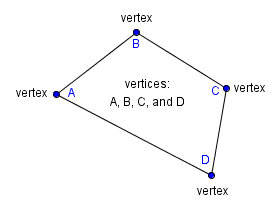NAMING POLYGONS
 When naming polygons, the vertices must be listed in consecutive order. For example, the polygon at right could be named: polygon $\,ABCD\,$ (start with $\,A\,$, move clockwise) polygon $\,ADCB\,$ (start with $\,A\,$, move counter-clockwise) polygon $\,BCDA\,$ (start with $\,B\,$, move clockwise) polygon $\,BADC\,$ (start with $\,B\,$, move counter-clockwise) polygon $\,CDAB\,$ (start with $\,C\,$, move clockwise) polygon $\,CBAD\,$ (start with $\,C\,$, move counter-clockwise) polygon $\,DABC\,$ (start with $\,D\,$, move clockwise) polygon $\,DCBA\,$ (start with $\,D\,$, move counter-clockwise)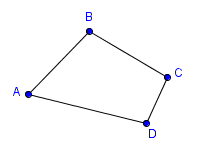More generally, when naming an $\,n$-gon, there are $\,n\,$ choices for listing the first vertex.
Then, there are $\,2\,$ choices for the next vertex (moving clockwise or counterclockwise).
The remaining vertices are then completely determined.
Thus, there are $\,2n\,$ choices for the polygon name.

DEFINITION regular polygon
A regular polygon is a polygon whose sides all have the same length,
and whose angles are all the same.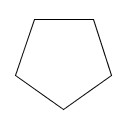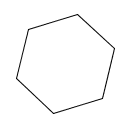a regular triangle a regular quadrilateral(a square) a regular pentagon a regular hexagon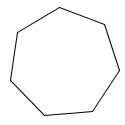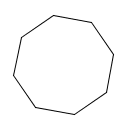a regular heptagon a regular octagon a regular nonagon a regular decagon
DEFINITIONS rectangle, square
A rectangle is a quadrilateral whose angles are all right angles.

A square is a rectangle with all sides of equal length.

Note: Every square is a rectangle.
However, not every rectangle is a square.
That is, there exist rectangles that are not squares.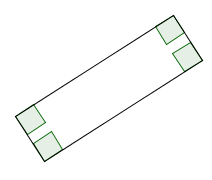each of these is a rectangle, but NOT a square

For fun, jump up to WolframAlpha and type in (say) ‘triangle’ or ‘quadrilateral’.

Master the ideas from this section